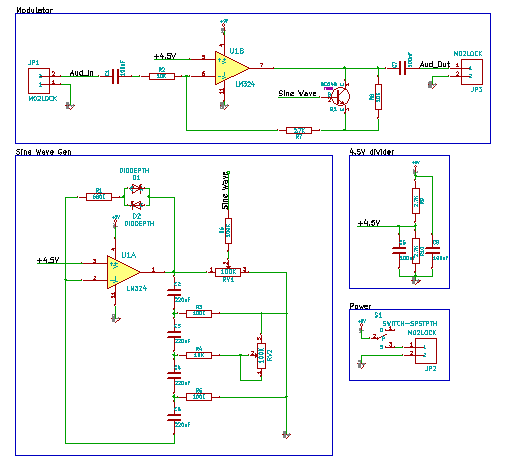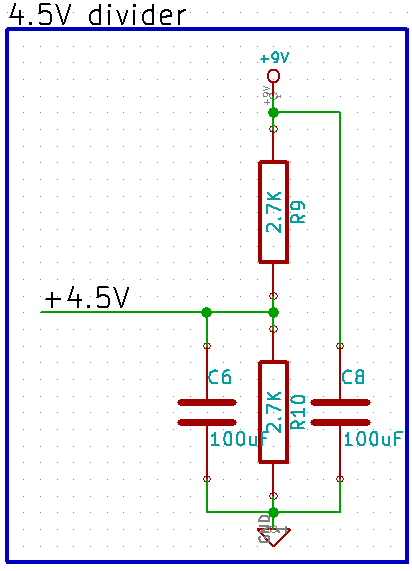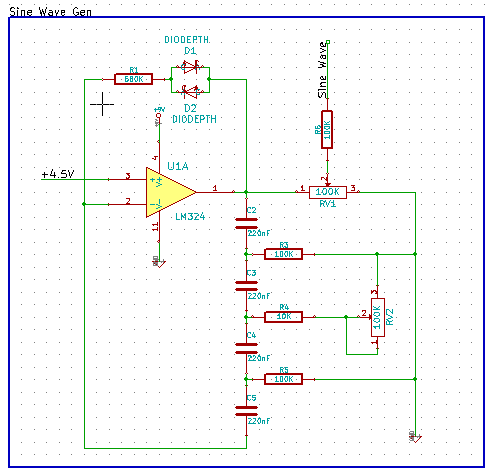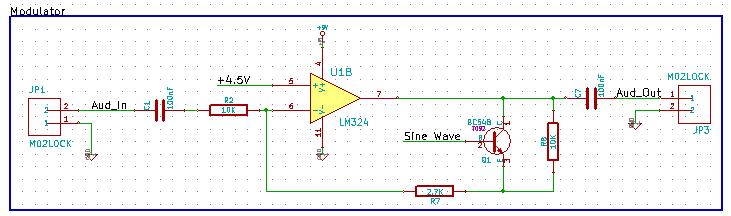Search Site

Tremolo Effects Pedal –

Building the circuit:

Ω      One LM324 Op-Amp

Ω      One BC548 Transistor

Ω      Two 100nF Capacitors

Ω      Two 100uF Capacitors

Ω      Four 220nF Capacitors

Ω      Three 100kΩ Resistors

Ω      Three 10kΩ Resistors

Ω      Three 2.7kΩ Resistors

Ω      One 680kΩ Resistor

Ω      Two 1n4001 diode

Ω      A breadboard, or PCB (which can be ordered from here.)

Ω      Some jumper cables, or solderAbove you can see the schematic for the project, but unlike the schematics we've seen before, it has been broken down into seperate blocks, to make it easier to understand. This is often done for more complex schematics as they would be incredibly messy without this.

Now for how it works:This is the power section, where the power comes into the schematic, through a switch.The next simplest bit is probably this bit, which is just a voltage divider to give us a nice 4.5V, and some smoothing capacitors across the supply rails. We need the 4.5V because this is what the signal will be centred on, and putting it directly in the middle of the supply rails makes it easier on the op-amp. Op-amps generally don't work as well closer to the supply rails, and most of the time don't output near them, to do this, you need a special kind of op-amp, referred to as a rail-to-rail op-amp.The next bit is the sine wave generator, this is a phase-shift oscillator configuration, with the op-amp for stability (without this op-amp if we were to try to draw any current out of the PSO the sine wave would collapse). RV1 and RV2 are potentiometers controlling the amount of modulation, and the frequency of modulation respectively.The final section is the modulator itself! This is the bit where the sine wave is used to modulate the signal from the instrument. The modulation is actually applied to the feedback loop of the op-amp with the transistor (BC548) the op-amp then applies this to the signal itself. The capacitor on the output is to block the DC component of the signal (the 4.5V) and leave the signal as just the amplitude modulated input.

If you want to see it working, here is a video: